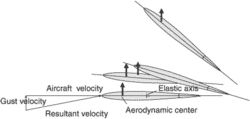# elastic axis

## elastic axis

[i′las·tik ′ak·səs]
(mechanics)
The lengthwise line of a beam along which transverse loads must be applied in order to produce bending only, with no torsion of the beam at any section.

## elastic axisIf an up-gust strikes the wing an increase on angle of attack results. Increased lift acts through the aerodynamic center. The wing twists nose upward about its elastic axis located some distance behind the aerodynamic center. This increases the angle of attack and lift even more and the self-perpetuation continues.
A span-wise line along the cantilever wing. Along this line, a load will produce bending but not torsion. The illustration shows an example of an elastic axis of a wing when it encounters gust. See also aileron reversal.
Mentioned in ?
References in classic literature ?
By this action, the highly elastic axis must be bent at the lower extremity, where it is naturally slightly curved; and I imagine it is by this elasticity alone that the zoophyte is enabled to rise again through the mud.
The motion of the profile is described by functions [alpha](t), representing the rotation around the elastic axis TR, and H(t), denoting the vertical displacement; see Figure 2.1.
The symbol F stands for the component of the aerodynamic force acting on the profile in the vertical direction, M is the torsional aerodynamic moment with respect to the elastic axis, [D.sub.HH], [D.sub.H[alpha]], [D.sub.[alpha]H], [D.sub.[alpha][alpha]], are the coefficients of structural damping, [S.sub.[alpha]], [I.sub.[alpha]], m and [k.sub.HH], [k.sub.[alpha][alpha]], [k.sub.H[alpha]], [k.sub.[alpha]H] denote the static moment around the elastic axis TR, the moment of inertia around TR, the mass of the profile and the stiffnesses of the profile elastic support.
[x.sub.1], [x.sub.2] are the coordinates of points on [[GAMMA].sub.Wt], [x.sup.TR.sub.i], i = 1, 2, are the coordinates of the elastic axis [x.sup.TR], and

Site: Follow: Share:
Open / Close